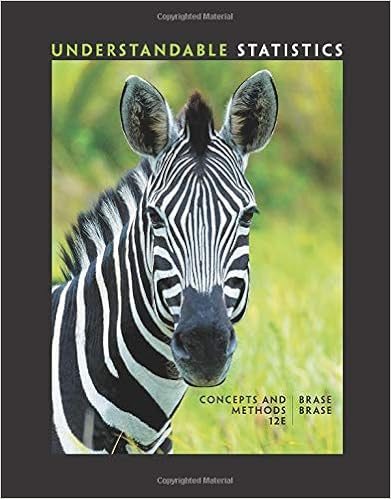# Assume the binomial distribution is appropriate if n

• 6
• 100% (2) 2 out of 2 people found this document helpful

This preview shows page 4 - 6 out of 6 pages.

##### We have textbook solutions for you!
The document you are viewing contains questions related to this textbook.The document you are viewing contains questions related to this textbook.
Chapter 3 / Exercise 7
Understandable Statistics: Concepts and Methods
BraseExpert Verified
17.Assume the binomial distribution is appropriate. If N= 6 and P= 0.50, the probability of getting exactly 5 events = _________.Pa.0.1094b.0.0156c.0.0609d.0.0938 – Correct Answer
##### We have textbook solutions for you!
The document you are viewing contains questions related to this textbook.The document you are viewing contains questions related to this textbook.
Chapter 3 / Exercise 7
Understandable Statistics: Concepts and Methods
BraseExpert Verified
18.Assume the binomial distribution is appropriate. If N= 10 and P= 0.30, the probability of getting at least 8 events is _________.P
19.Suppose you gave a multiple-choice exam with 16 questions on it. Each question has 4 alternatives. What is the probability that a student who guesses at random on each of the 16 questions will score 4 or fewer correct answers? Assume that each alternative to each question is equally likely to be chosen.
20. (2 points) In a population survey of patients in a rehabilitation hospital, the mean length of stay in the hospital was 12.0 weeks with a standard deviation equal to 1.0 week. The distribution was normally distributed. What is the percentile rank for each of the following z scores? Enter the percentile rank to the right of each z score. Assume a normal distribution.
_b) 0.89 ___
21. (4 points). The incidence of eating disorders in the general population is .20. Many factors are taken into account like your score on the Eating Disorders Inventory. Previous work suggests that out of those people diagnosed with Eating Disorders, .70 of them score above 40 on the EatingDisorders Inventory (EDI) while .20 of those were not diagnosed with eating disorders scored above 40 on the EDI. What is the probability of being diagnosed with an Eating Disorder given that you scored above 40 on the EDI? Does giving the EDI improve the diagnostic accuracy for eating disorders?
.2
47Does giving the EDI improve the diagnostic accuracy for eating disorders? Why or Why not?
•••